QuestionAnswers

# What is the name of a parallelogram with all sides equal? \begin{align} & \text{a) Square} \\ & \text{b) Rhombus} \\ & \text{c) Rectangle} \\ & \text{d) Trapezium} \\ \end{align}

Hint: Now any figure with 4 sides is called Quadrilateral. In a parallelogram opposite sides are equal and parallel. Also the opposite angles of parallelogram are equal.

Any figure which has 4 sides and 4 vertices is called a Quadrilateral.
Now each different Quadrilateral has its own definition.
A Parallelogram is a Quadrilateral with its parallel side equal and opposite as well as its opposite angles are equal.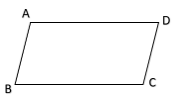A Square is Quadrilateral with opposite sides parallel, all sides equal and all the angles are equal.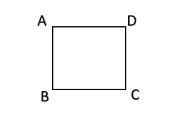A Rhombus is a Quadrilateral with opposite sides parallel and all sides equal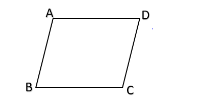A Rectangle is a Quadrilateral with all opposite sides equal and parallel and all the angles are equal.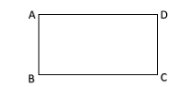A Trapezium is a quadrilateral with one pair of opposite sides parallel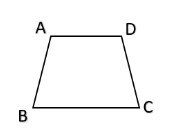Now, Consider a Parallelogram with all sides equal.
If all its sides are equal we get a parallelogram with opposite sides parallel and opposite angles equal as well as all its sides are also equal.
Now we know that a Quadrilateral with all its side equal and opposite angles also equal is a Rhombus.
Hence, the name of the parallelogram with all sides equal will be Rhombus.
Now since the angles are not all equal the Quadrilateral cannot be a Square or Rectangle.
Also since both opposite sides are parallel the Quadrilateral is not a Trapezium.
So, the correct answer is “Option B”.

Note: Rhombus can sometimes be confused with square since both the quadrilaterals have a common property that all its sides are equal. However the thing that distinguishes a Rhombus from a square is the property that all the angles of the square are also equal and in fact each angle is equal to 90° while in Rhombus just the opposite angles are equal. Hence, we can say that every square is a Rhombus but every Rhombus need not be a square.